## Introduction to Sensitivity Analysis

We apply Sensitivity Analysis to a financial model to determine how different values of an independent variable affect a specific dependent variable under a given set of assumptions.

We also refer to it as ‘what-if’ or simulation analysis. Performing such analysis helps us predict better the outcome of a decision, based on a range of variables.

Sensitivity Analysis is instrumental in ‘black-box’ situations, where the output is the result of a multi-step complex formula of more inputs, making it impossible to analyze.

We can apply the concept of Sensitivity Analysis in a vast number of cases within forecasting and modeling.

By analyzing both the target and input variables, we can look at how the variables move and how one affects the other. Based on this, we can create better ranges for the sensitivity analysis of those variables

Sensitivity analysis should not be confused for scenario analysis. Sensitivity analysis looks into understanding the relationship between input and target variables, while scenario analysis requires describing a specific scenario in detail. Also, sensitivity analysis looks at the effect of isolated changes in inputs, while scenario analysis looks at situations of significant changes. Usually, we would use scenario and sensitivity analysis together to achieve a more comprehensive understanding of the possible outcomes.

## How To Analyze Sensitivity

The principle behind sensitivity analysis is based on changing one input in the model and observing the changes in model behavior.

To perform sensitivity analysis, we follow these steps:

1. Define the base case of the model;
2. Calculate the output variable for a new input variable, leaving all other assumptions unchanged;
3. Calculate the sensitivity by dividing the % change in the output variable over the % change in the input variable.

We then repeat those three steps to get the output’s sensitivity to each of the independent variables. The higher the calculated sensitivity metric, the more sensitive the output is to changes in this input. We refer to this approach as ‘local sensitivity analysis,’ or a One-At-a-Time (OAT) analysis.

The other popular approach is ‘global sensitivity analysis,’ usually implemented with Monte Carlo simulation techniques.

Sensitivity analysis is a widely-adopted technique due to the many benefits that it brings to the table:

(+) Acts as an in-depth analysis and study of the variables and their behavior;

(+) Usually leads to more reliable predictions;

(+) Helps decision-makers identify where to improve in future iterations of the financial model;

(+) Adds credibility to any economic model by testing it across a wide range of possibilities;

(+) Shows how responsive the output is to changes in specific values.
There are also some disadvantages to relying on sensitivity analysis:

(-) Outcomes are all based on assumptions on top of historical data, which makes them susceptible to being incorrect;

(-) Looking at each factor individually, doesn’t allow us to analyze the interaction and correlation between variables, as well as any effect it may have.

## Sensitivity Analysis Application

An essential application of Sensitivity Analysis is in the models prepared by managers and decision-makers. Sensitivity analysis also helps us understand the uncertainties, limitations, and scope of the decision model. After all, we make most decisions under uncertainty, so it’s beneficial to know how much impact can different independent variables have on the dependent variable.

We can also use the approach as an auditing tool for financial models. It can help us identify errors in the model, which makes it beneficial for risk analysis as well.

## Performing Sensitivity Analysis in Excel

To better understand how Sensitivity Analysis can improve our financial models, let us look at one of the most common ways to apply it.

We are valuing a potential investment in a company with the following historical data for the past three years:We have also made the following assumptions for our model: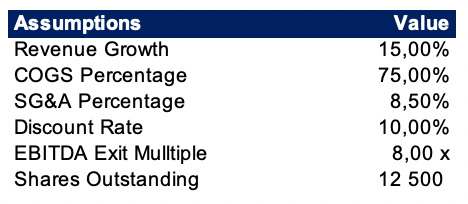Applying the assumptions for Revenue Growth, COGS Percentage, and SG&A Percentage, we can build a simple forecast for the next five years.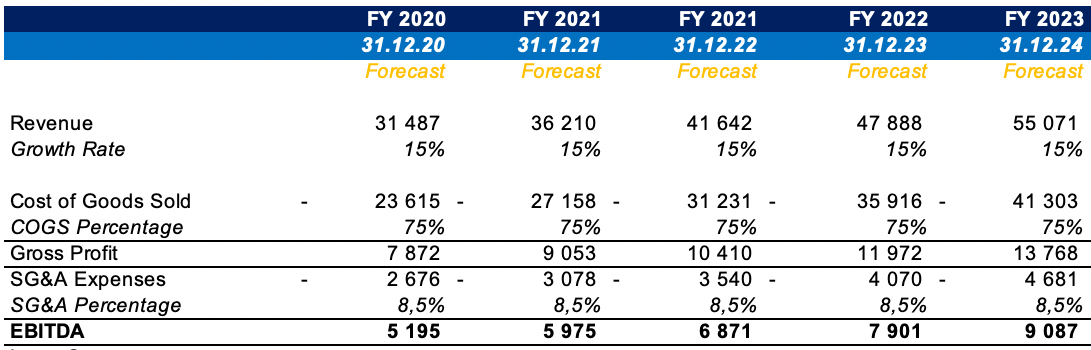Assuming no Capex and the following Net Working Capital changes, we arrive at the resulting Free Cash Flow for the five years.

We also add another Exit period for the terminal value, that we can calculate based on last period EBITDA and the EBITDA multiple from the assumptions we made earlier.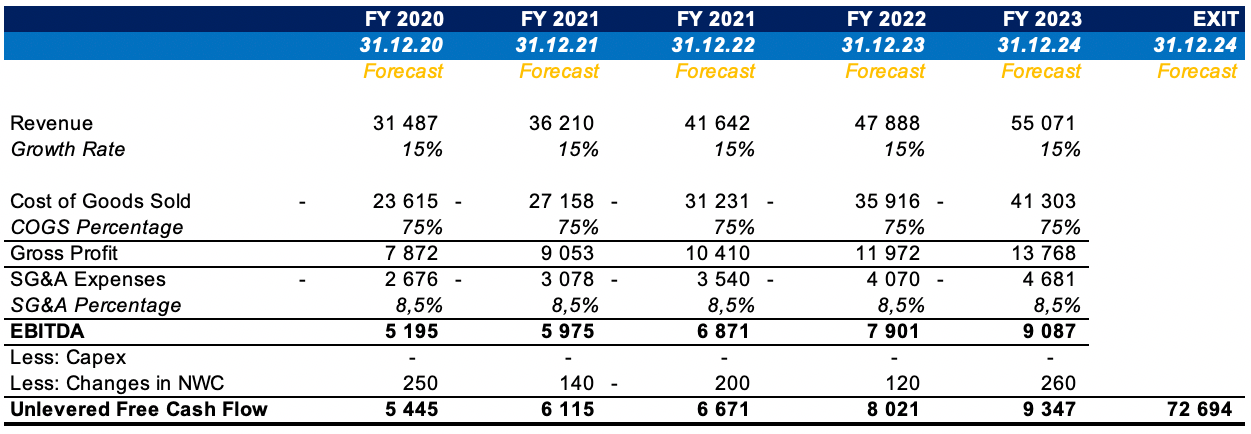We can then use the XNPV formula in Excel to calculate the Net Present Value of the Free Cash Flow for the period.Taking the NPV of our forecasted cash flows as the Enterprise Value, we can add cash and subtract debt to arrive at the Equity Value. Dividing this over the 12,500 outstanding shares, we arrive at an Equity Value per Share of EUR 6.26 thousand.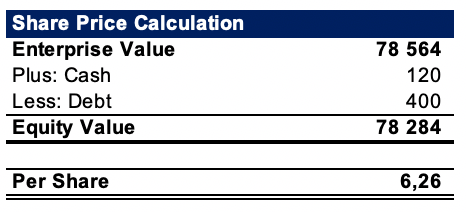Let us prepare a table for our sensitivity analysis. We will be looking into how changes in EBITDA multiple and Revenue Growth will affect the share price calculation.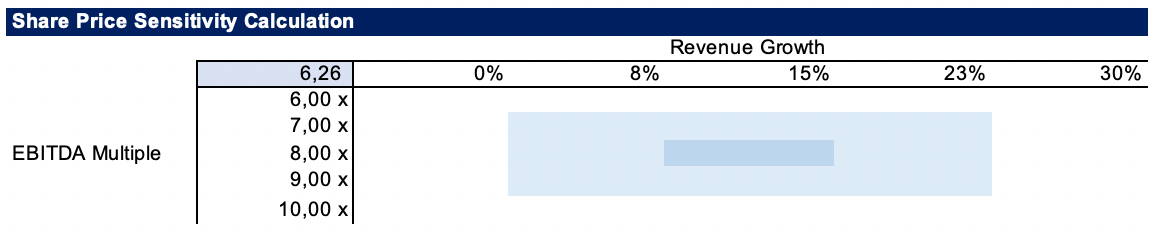After we have the table set-up in this way, with the calculated Share Price linked in the top-left cell, we can use the Data Table functionality of Excel, under the Data tab, in the What-If Analysis drop-down.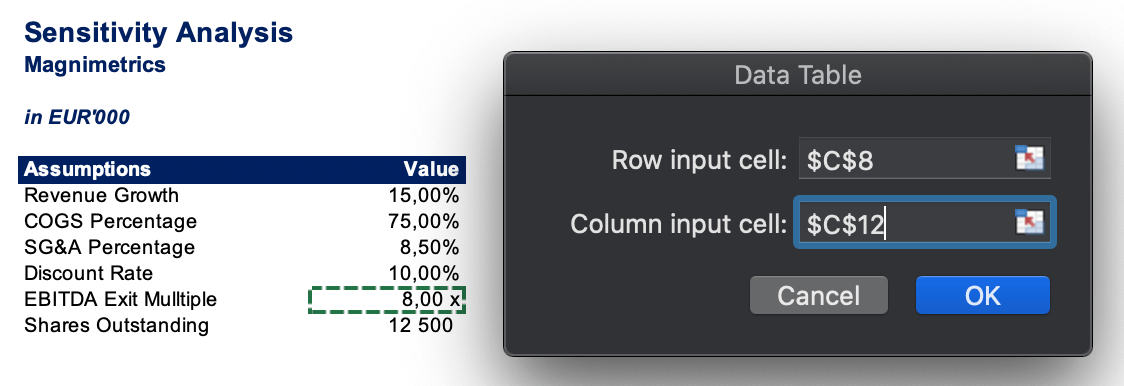Basically the idea is that we select the two input variables (growth rate of revenue and EBITDA multiple), and the data table calculates the output variable (share price) per each combination of values of the input variables.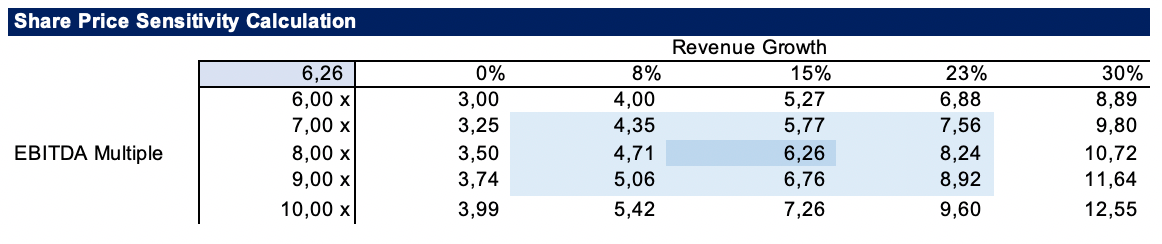Having this table, we can then perform a much more thorough analysis and support a better decision-making process. It is instrumental to analyze how the share price of the potential investment will be affected by possible changes in revenue growth and the exit EBITDA multiple. Being able to provide ranges for the independent variables, within which we can expect a positive return will ultimately aid us in making a more informed decision.

## Conclusion

Sensitivity analysis is an instrumental tool in the arsenal of any financial analyst. It provides insights into the problems with the model. It also gives us an idea about how sensitive is the optimum solution chosen to variations in the input values of one or more independent variables.## FCCA, FMVA

Hi! I am a finance professional with 10+ years of experience in audit, controlling, reporting, financial analysis and modeling. I am excited to delve deep into specifics of various industries, where I can identify the best solutions for clients I work with.

In my spare time, I am into skiing, hiking and running. I am also active on Instagram and YouTube, where I try different ways to express my creative side.